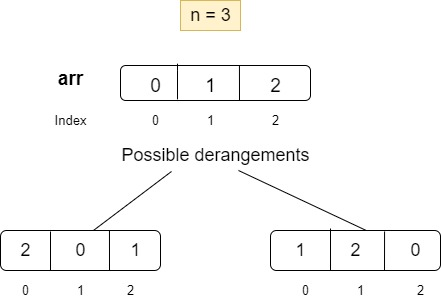1

# Count derangements

Difficulty: MEDIUM
Avg. time to solve
35 min
Success Rate
60%

Problem Statement
Suggest Edit

#### Given a number ‘n’, find the total number of derangements possible of a set of ‘n’ elements.

##### Note:
``````The answer could be very large, output answer%(10^9+7).
``````
##### Input format:
``````The first line of input contains an integer ‘T’ denoting the number of test cases.
The next ‘T’ lines represent the ‘T’ test cases.

The first line and the only line of each test case contains an integer ‘n’ denoting the number of elements whose derangements are to be counted.
``````
##### Output Format:
``````For each test case, return the total number of derangements of a set of ‘n’ elements.
``````
##### Constraints:
``````1 <= T <= 100
1 <= n <= 3000

Where ‘T’ is the total number of test cases, ‘n’ denotes the number of elements whose derangements are to be counted.

Time limit: 1 second
``````
##### Sample Input 1:
``````2
2
3
``````
##### Sample Output 1:
``````1
2
``````
##### Explanation of sample input 1:
``````There are two total test cases as represented by the first line.

Test case1: For two elements say {0, 1}, there is only one possible derangement {1, 0}. 1 is present at index 0 and not at its actual position, that is, 1. Similarly 0 is present at index 1 and not at its actual position, that is, 0.

Test case 2: For three elements say {0, 1, 2}, there are two possible derangements {2, 0, 1} and {1, 2, 0}. In both the derangements, no element is present at its actual position.
``````##### Sample Input 2:
``````2
5
4
``````
##### Sample Output 2:
``````44
9
``````Want to solve this problem? Login now to get access to solve the problems